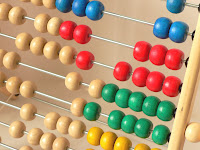Percents show up a lot in the GRE quantative section. You can really speed yourself up by being able to figure them quickly in your head. That translates to more time on the tough problems.

Can you find 18% of 246 in your head? Here’s a good way to do it. First, some basics. Most of us can quickly figure out what ten percent of a number is. Just move the decimal one space to the left. For example, what’s 10% of 246? Think of it as 246.0. Now just move the decimal one space to the left to get 24.6. More examples,

10% of …

47 is 4.7
1,433 is 143.3
10,012 is 1,001.2

Easy enough. You can do 1% of a number just as easily. Just move the decimal 2 spaces left. So 1% of 246 would be 2.46. We took 246.0 and moved the decimal 2 spaces to the left.

1% of …

47 is .47
1,433 is 14.33
10,012 is 100.12

Finding 5% of a number is easy too. Just find 10% and divide it in half. So,

5% of …

47 is 2.35
10,012 is 500.6

Finding 5% may take a little practice before you can do it reliably in your head. Practice for twenty minutes or so, and you’ll have it down.

Now how do we find 18% of something? Just think of 18% as 10% + 5% + 1% + 1% + 1%. Let’s find 18% of 246.

10% of 246 is 24.6
5% is half that 12.3
so 15% … 24.6 + 12.3 is 36.9 (remember that)
1% would be 2.46, but I have three of these so I add 2.46 plus 2.46 … errr … too tough. Let’s just add 2.5 to 2.5 which is close enough. That’s 5. Plus another 2.5 is 7.5.

So 36.9 + 7.5 = 44.4 . Your calculator will return 44.28, so we were pretty close.

Again, it will take some practice to be able to hold the numbers in your head while you add them all up, and some numbers are easier than others, but this method is really powerful. A little practice will pay big dividends!

Here are some to practice on. Remember, this is estimating, so just get pretty close.

1. 27% of 82
2. 43% of 118
3. 98% of 489
4. 77% of 1500
5. 12% of 1228

Here are the approximate answers …

1. 22.14
2. 51.5
3. 479
4. 1155 (I did this one as 75% of 1000 is 750 and half of that would be 75% of 500, so 375. 750 + 375 is 1,125 plus two one percents–30–is 1,155).
5. 146

Categories:

Tags: